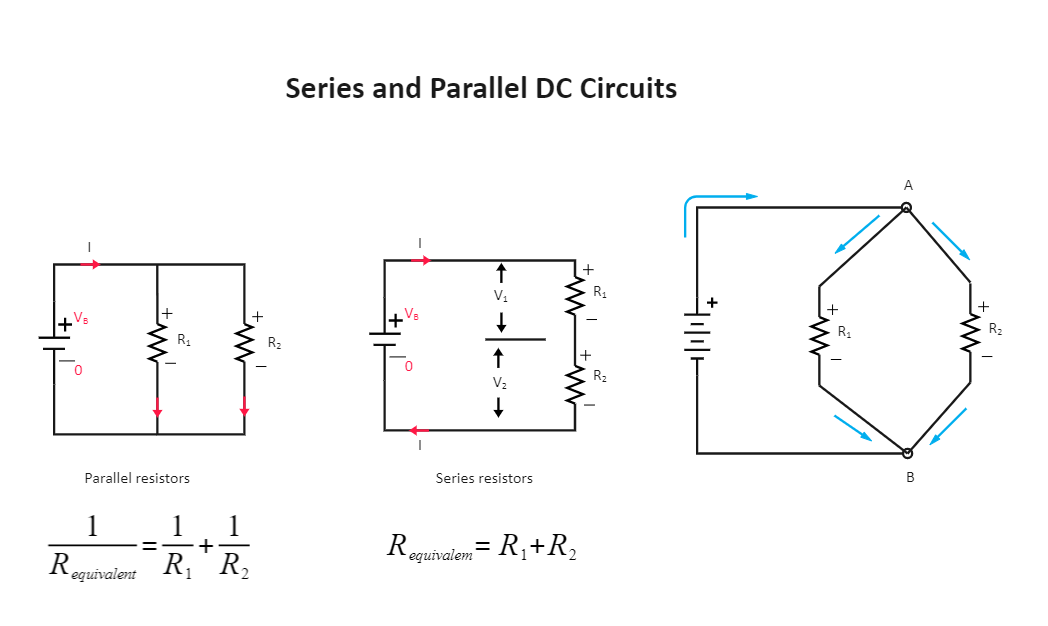Template Community / Series Circuit Diagram

# Series Circuit DiagramBlackwood
Published on 2022-03-23
Edit OnlineIntroduction

A DC or direct current circuit is one where the current flows only in a single direction. Majorly all electrical circuits require a DC power source. In the case of an open DC circuit, there is no current flow. Vice versa, in a closed DC circuit, there is power flow throughout the device. A switch functions the role of an open and closed circuit here. Through the Series Circuit Diagram, let's learn its function and components.

1. Understanding Series Circuit Diagram

The key components in a DC supply are majorly resistors. When connected in series, they are called series DC circuits, and when connected in parallel, they are referred to as parallel DC circuits.

In case we have three resistors, and they are connected in series, it is a series circuit diagram. These resistors are connected across a voltage source, and current flows through the single path. The current flow across all resistors is the same in a series connection.
In cases when the resistors are connected in parallel with each other, they are called parallel circuit diagrams. Same as a series circuit, resistors are connected across a voltage source, and current flows through the single path in a parallel circuit. In this circuit, the voltage drop across each resistor is the same.
DC circuits have different types, namely series, parallel, and series-parallel. In a series-parallel circuit, the resistors are connected in parallel to each other, and they are also in series with other resistors.

2. How to Create a Series Circuit Diagram using EdrawMax Online?

Creating a Series Circuit Diagram in EdrawMax Online is pretty simple. The free Electrical Circuit maker has several features as you can instantly import the images or icons from your system, Google Drive, or DropBox. Your Electrical Circuit diagram will require additional media content, making it more creative.

Choose a template
EdrawMax Online comes with hundreds of free diagram templates. Select a pre-designed template by entering the Keyword in the "Search" section or exploring different diagram sets. In this case, you will find different types of Electrical Circuits diagrams under the "Electrical Engineering" section under the "Engineering" section. You will find different diagram sets, like Basic Electrical, Circuits and Logic, Industrial Control System, and Systems' Diagram. Alternatively, you can simply click on "+" in EdrawMax Online canvas to create a diagram of your preference.

The Series Circuit Diagram below states that a series circuit has more than one resistor but only one path through which the electricity (electrons) flows. From one end of the cell (battery), the electrons move along one line with no branches, through the resistors, to the other end of the cell.

Customize the diagram
Customize your Series Circuit diagrams by changing the color or adding more relevant data. Based on your research, you can also add or remove the Electrical Circuit symbols or content accordingly. Since it is about electrical engineering research, you can add more relevant data about different circuit diagrams.

Export & Share
Once your required circuit diagram is completed, you can share it amongst your colleagues or clients using the easy export and share option. You can further export the diagram in multiple formats, like Graphics, JPEG, PDF, or HTML. Also, you can share the designs on different social media platforms, like Facebook, Twitter, LinkedIn, or Line. In simple words, you can export your incredible Series Circuit diagrams to the files you want with just a few clicks.

3. Important Points to Consider While Creating Series Circuit Diagram

Since there are different DC circuits, like series and parallel, the connections must be made thoroughly.
In the case of a series connection, make sure to connect all resistors in series with each other.
In the parallel circuit, ensure to connect all resistors in parallel.
Make sure to connect the voltage supply at the input side
Mark the flow of current and voltage throughout the system or the circuit.
The number of resistors used can depend on the output.
If you are using more than one resistor, mark it as 1, 2, and so on.

Conclusion
The DC circuit, direct current circuit, or Series Circuit Diagram has wide applications and is used in many electronic devices. The current flows through the circuit in a closed path and generates an output signal—the open-circuit acts as an off switch, preventing current flow through the system or the device. It is recommended to use EdrawMax Online to create similar-looking circuit diagrams for your projects. It should be noted here that since these circuit diagrams are the blueprints of the actual working models, ensure that you use the correct symbols provided by EdrawMax Online.
Tag
circuit diagram
electrical plan
Circuit Diagram Collection
Report
1
101PostRecommended Templates